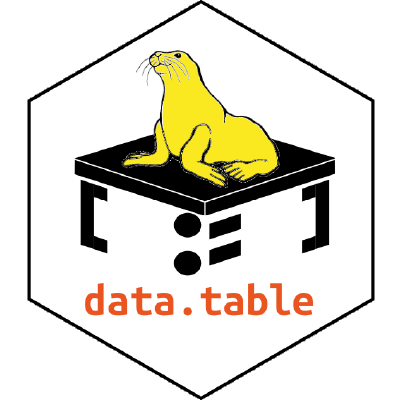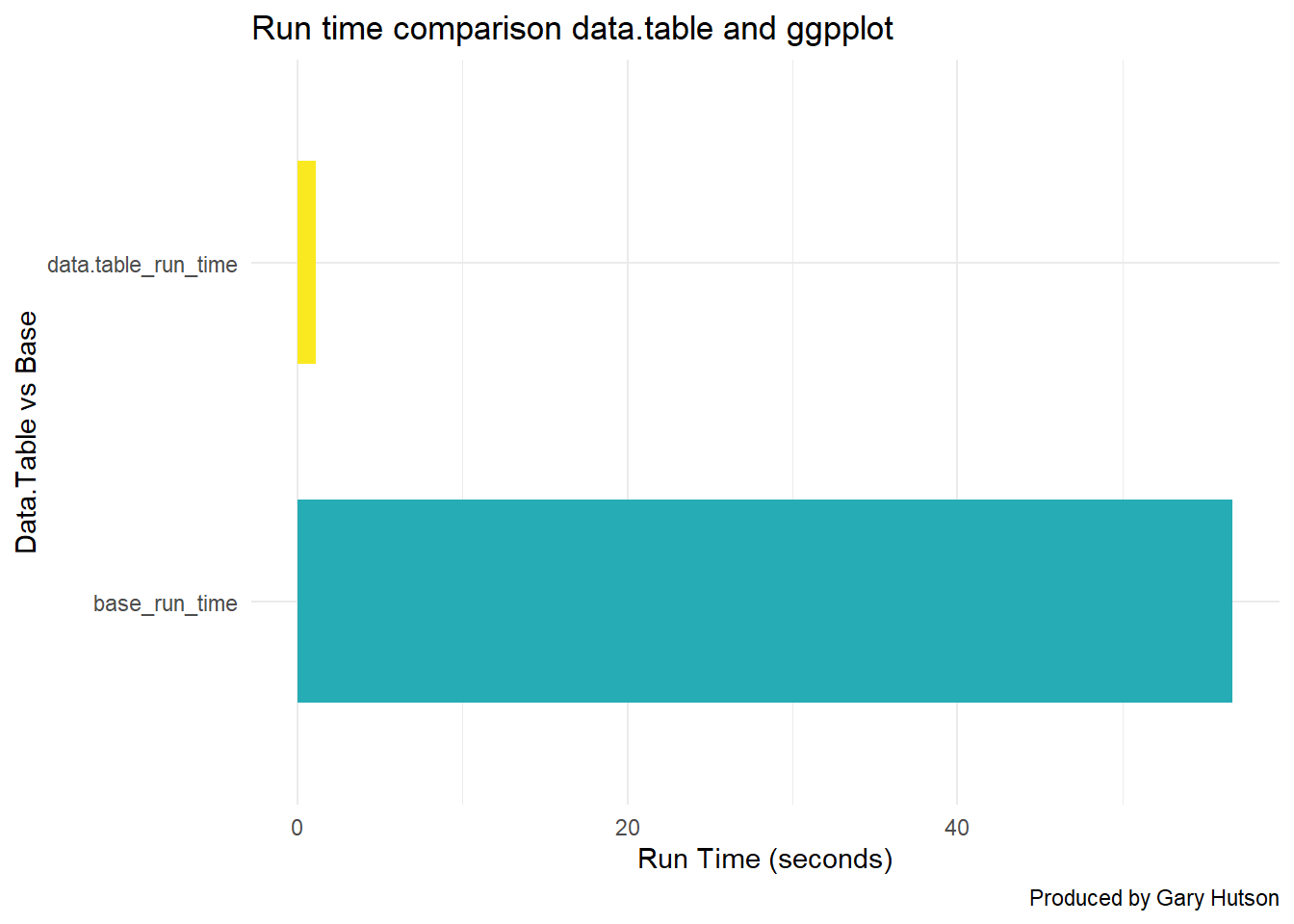## Data.Table - everything you need to know to get you started in R

I will take you through step by step how to use the data.table package, and compare it with base R operations, to see the performance gains you get when using this optimised package.

## Load in data.table

To load the package in you can follow the below instructions:

``````#install.packages(data.table)
library(data.table)``````

### Reading in a data.table csv

To read files in data.table you use the fread syntax to bring files in. I will load the NHSRDatasets package and export this out and then I will use the data.table functionality to read it back in:

``````library(NHSRdatasets)
ae <- NHSRdatasets::ae_attendances
write.csv(ae, "ae_nhsr.csv", row.names = FALSE) #Set row names to false
#Use data.table to read in the document
ae_dt <- fread("ae_nhsr.csv")``````

## Benchmarking the speed of data.table vs base R

Here, we will create a synthetic frame, using a random number generator, to show how quick the data.table package is compared to base R:

``````#Create a random uniform distribution
big_data <- data.frame(BigNumbers=runif(matrix(10000000, 10000000)))
write.csv(big_data, "bigdata.csv")``````

Start the benchmarking:

``````# Start to benchmark using system.time
# Read CSV with base
base_metrics <- system.time(
read.csv("bigdata.csv")
)

dt_metrics <- system.time(
data.table::fread("bigdata.csv")
)
print(base_metrics)``````
``````##    user  system elapsed
##   56.69    1.33   58.95``````
``print(dt_metrics)``
``````##    user  system elapsed
##    1.11    0.21    0.38``````
``````# # user  system elapsed
#   25.78    0.42   26.74
#    user  system elapsed
#    1.09    0.07    0.33 ``````

Compare on a ggplot side by side:

``library(dplyr)``
``````##
## Attaching package: 'dplyr'``````
``````## The following objects are masked from 'package:data.table':
##
##     between, first, last``````
``````## The following objects are masked from 'package:stats':
##
##     filter, lag``````
``````## The following objects are masked from 'package:base':
##
##     intersect, setdiff, setequal, union``````
``````library(tibble)
library(ggplot2)

df <- data.frame(
base_run_time = base_metrics, #Grab the elapsed time for the user
data.table_run_time = dt_metrics #Grab the elapsed time for the user
)
#Flip data.frame over to get the run times using transpose
df<- data.frame(t(df)) %>%
rownames_to_column() %>%
setNames(c("Type", "TimeRan"))

# Make the ggplot
library(ggplot2)

plot <- df %>%
ggplot(aes(x=Type,
y=TimeRan,
fill=as.factor(Type))) + geom_bar(stat="identity",
width = 0.6) +
scale_fill_manual(values= c("#26ACB5", "#FAE920")) + theme_minimal() +
theme(legend.position = "none") + coord_flip() +
labs(title="Run time comparison data.table and ggpplot",
y="Run Time (seconds)",
x="Data.Table vs Base",
caption="Produced by Gary Hutson")

print(plot)``````As you can see - data.table is lightening fast compared to base R and it is great for working with large datasets.

We detract, this section is just to highlight how useful the data.table package is for dealing with larger datasets.

## Conversion between data.table and data.frame (base) objects

Time to time you may want to convert the data.table objects back to base R, to do this you can follow the below:

``````#Convert base data.frame to data.table
ae_dt <- as.data.table(ae)
class(ae_dt)``````
``##  "data.table" "data.frame"``
``````#Using the setDT command
ae_copy <- ae
data.table::setDT(ae_copy)
class(ae_copy)``````
``##  "data.table" "data.frame"``
``````# Converting this back to a data.frame
data.table::setDF(ae_copy)
class(ae_copy)``````
``##  "data.frame"``
``````#  "data.table" "data.frame"
#  "data.table" "data.frame"
#  "data.frame"``````

To expand on the above:

• I set the original A and E data, from the loading example, using the as.data.table command, this coerced the data.frame into a data.table object. We inspect that this has been changed by checking the class of the object
• I then made a copy of the data.frame and used the setDT() syntax to set it to a data.table object. Again, I then used class to check the class of the object
• Finally, I used the setDF to force it back to a data.frame, as this object had been converted to a data.table object in the previous step. I used class to check the type and this has successfully been changed back.

## Filtering on a data.table object

The general rule to filtering is to use the below visual: